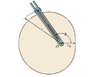# Dynamics - Cylindrical Coordinates

• sami23

## Homework Statement

A cam has a shape that is described by the function r = r_0(2 - cos $$\theta$$), where r_0 = 2.25 ft. A slotted bar is attached to the origin and rotates in the horizontal plane with a constant angular velocity ($$\dot{\theta}$$ dot) of 0.85 radians/s. The bar moves a roller weighing 25.6 lb along the cam's perimeter. A spring holds the roller in place; the spring's spring constant is 1.75 lb/ft. The friction in the system is negligible. When $$\theta$$ = 102 degrees, what are F_r and F_$$\theta$$, the magnitudes of the cylindrical components of the total force acting on the roller?

## Homework Equations

r = r_0(2 - cos $$\theta$$) = 2.25(2-cos102) = 4.9678 m
$$\dot{\theta}$$ = 0.85 rad/s
$$\ddot{\theta}$$ = 0 rad/s2
$$\dot{r}$$ = (r_0sin$$\theta$$)($$\dot{\theta}$$) = 2.25(.85)sin(102) = 1.87 m/s
$$\ddot{r}$$ = (r_0cos$$\theta$$)($$\dot{\theta}$$)^2 + (r_0sin$$\theta$$)($$\ddot{\theta}$$) = 2.25(.852)cos(102) = -0.338 m/s2

a_r = $$\ddot{r}$$ - r($$\dot{\theta}$$)^2
a_$$\theta$$ = r$$\theta$$ + 2$$\dot{r}$$$$\dot{\theta}$$

F_r = W/g * a_r
F_$$\theta$$ = W/g * a_$$\theta$$

F_s = ks (spring)

## The Attempt at a Solution

a_r = $$\ddot{r}$$ - r($$\dot{\theta}$$)^2 = -0.338 - 4.9678(.85^2) = -3.927 m/s2

a_$$\theta$$ = r$$\theta$$ + 2$$\dot{r}$$$$\dot{\theta}$$ = 4.9678*0 + 2*1.87*.85 = 3.179 rad/s2

F_r = W/g * a_r = (25.6/32.2)*(-3.927) = 3.12 lb
F_$$\theta$$ = W/g * a_$$\theta$$ = (25.6/32.2)*(3.179) = 2.53 lb

But it's wrong and I didn't consider the spring force. How do I incorporate Fs? Please help. I'm stuck

#### Attachments

•cam.jpg
7 KB · Views: 403
Last edited:
it opposes the acceleration along r

Last edited:
I found F_r = -3.122 lb and F_$$\theta$$ = 2.531 lb when a_r = -3.927 rad/s2 and a_$$\theta$$ = 3.179 rad/s2

What are F_t and N, the magnitudes of the tangential force, F_t, and the normal force, N, acting on the roller when $$\theta$$ = 102 degrees?
a = a_t + a_n

a_t = dv/dt (angular velocity)
a_n = v2/$$\rho$$ where $$\rho$$ = radius of curvature

Equations of Motion:
F_t = W/g * a_t
F_n = W/g * a_n where g = 32.2 ft/s2

How do I find a_t and a_n to get the tangential force and the Normal? Please help.